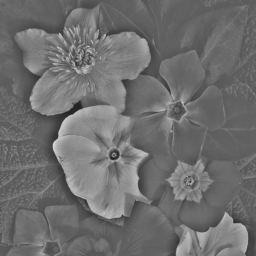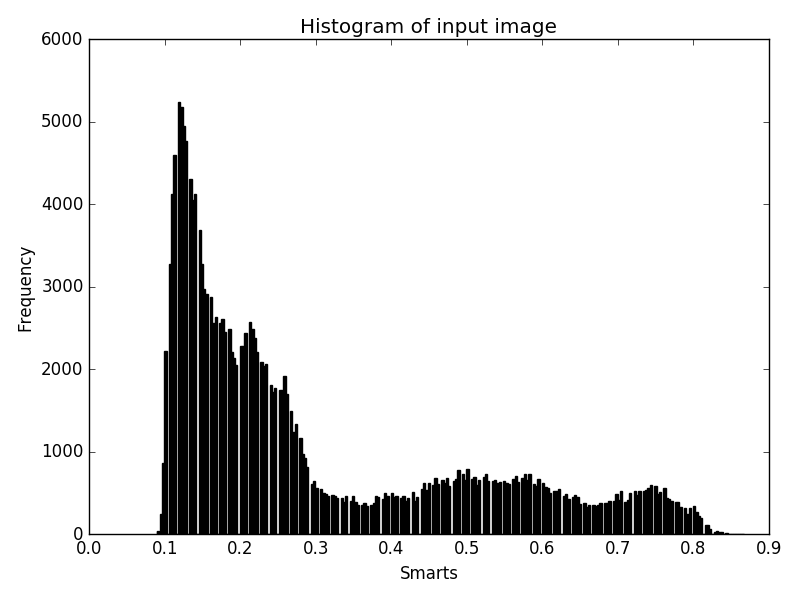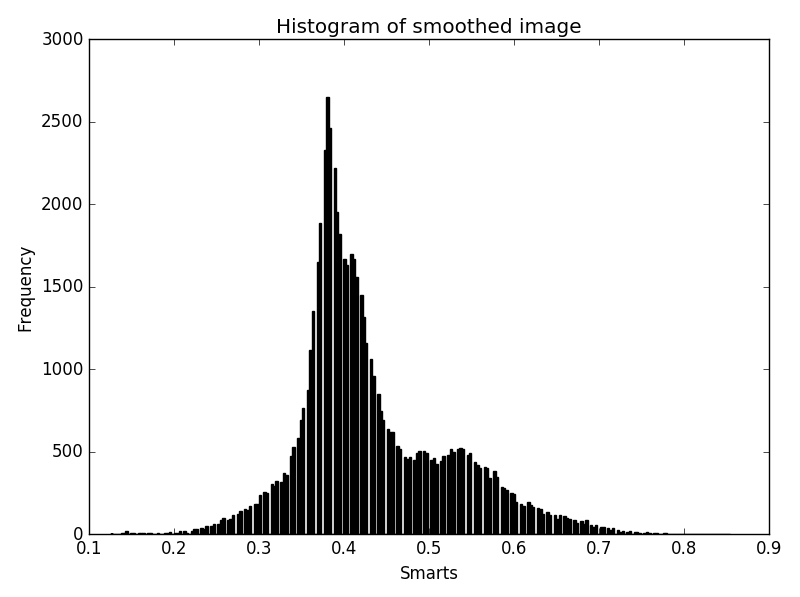# Adaptive Histogram Equalization Image Filter#

## Synopsis#

Apply a power law adaptive histogram equalization controlled by the parameters alpha and beta.

The parameter `alpha` controls how much the filter acts like the classical histogram equalization method (`alpha = 0`) to how much the filter acts like an unsharp mask (`alpha = 1`).

The parameter `beta` controls how much the filter acts like an unsharp mask (`beta = 0`) to much the filter acts like pass through (```beta = 1, with alpha = 1```).

The parameter window (or radius) controls the size of the region over which local statistics are calculated.

## Results#Input image. Output image.Input image histogram. Output image histogram.

## Code#

### C++#

```#include "itkAdaptiveHistogramEqualizationImageFilter.h"
#include "itkImageFileWriter.h"

int
main(int argc, char * argv[])
{
if (argc < 6)
{
std::cerr << "Missing parameters." << std::endl;
std::cerr << "Usage: " << argv << " inputImageFile outputImageFile alpha beta radius" << std::endl;
return EXIT_FAILURE;
}

constexpr unsigned int Dimension = 2;

using PixelType = unsigned char;
using ImageType = itk::Image<PixelType, Dimension>;

float alpha = std::stod(argv);

float beta = std::stod(argv);

return EXIT_SUCCESS;
}
```

### Python#

```#!/usr/bin/env python

import itk
import argparse

parser = argparse.ArgumentParser(
)
args = parser.parse_args()

Dimension = 2

PixelType = itk.ctype("unsigned char")
ImageType = itk.Image[PixelType, Dimension]

histogramEqualization.SetAlpha(args.alpha)
histogramEqualization.SetBeta(args.beta)

itk.imwrite(histogramEqualization, args.output_image)
```

## Classes demonstrated#

template<typename TImageType, typename TKernel = Neighborhood<bool, TImageType::ImageDimension>>

Histogram equalization modifies the contrast in an image. The AdaptiveHistogramEqualizationImageFilter is a superset of many contrast enhancing filters. By modifying its parameters (alpha, beta, and window), the AdaptiveHistogramEqualizationImageFilter can produce an adaptively equalized histogram or a version of unsharp mask (local mean subtraction). Instead of applying a strict histogram equalization in a window about a pixel, this filter prescribes a mapping function (power law) controlled by the parameters alpha and beta.

The parameter alpha controls how much the filter acts like the classical histogram equalization method (alpha=0) to how much the filter acts like an unsharp mask (alpha=1).

The parameter beta controls how much the filter acts like an unsharp mask (beta=0) to much the filter acts like pass through (beta=1, with alpha=1).

The parameter window controls the size of the region over which local statistics are calculated. The size of the window is controlled by SetRadius the default Radius is 5 in all directions.

By altering alpha, beta and window, a host of equalization and unsharp masking filters is available.

The boundary condition ignores the part of the neighborhood outside the image, and over-weights the valid part of the neighborhood.

For detail description, reference “Adaptive Image Contrast

Enhancement using Generalizations of Histogram Equalization.” J.Alex Stark. IEEE Transactions on

Image Processing, May 2000.

ITK Sphinx Examples: